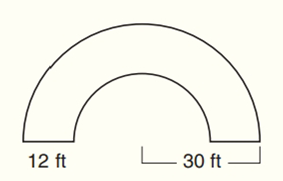Chapter 8.CR, Problem 40CRElementary Geometry For College St...

7th Edition
Alexander + 2 others
ISBN: 9781337614085

Solutions

Chapter
SectionElementary Geometry For College St...

7th Edition
Alexander + 2 others
ISBN: 9781337614085
Textbook Problem

Sue and Dave’s semicircular driveway is to be resealed, and then flowers are to be planted on either side.(Give approximate answers.)a) What is the number of square feet to be resealed?b) If Dave can reseal the driveway at a cost of \$0.45 per square feet, what is the cost of resealing the driveway?c) If individual flowers are to be planted 1 foot from the edge of the driveway at intervals of approximately 1 foot on both sides of the driveway, how many flowers are needed?To determine

(a)

To find:

The number of square feet to be resealed.

Explanation

Formula:

The area of the semi-circle A=12πr2.

Calculation:

Consider the following diagram:

We need to find the number of square feet to be resealed.

We determine the area of ring:

OB=ODBD=ODAC=3012=18

Aring=Abig semi-circleAsmall semi-circle=π(OD2)2π<

To determine

(b)

To find:

The cost of resealing the driveway.

To determine

(c)

To find:

The number of flowers are needed to be planted 1 foot from the edge of the driveway.

Still sussing out bartleby?

Check out a sample textbook solution.

See a sample solution

The Solution to Your Study Problems

Bartleby provides explanations to thousands of textbook problems written by our experts, many with advanced degrees!

Get Started

In Exercises 1-4, simplify the expression. 2x22x124x224x+36

Calculus: An Applied Approach (MindTap Course List)

If f and g are increasing on an interval I, then f g is increasing on I.

Single Variable Calculus: Early Transcendentals, Volume I

Other Equations Find all real solutions of the equation. 108. x3x44=0

Precalculus: Mathematics for Calculus (Standalone Book)

In Problems 1-6 simplify each fraction. 6.

Mathematical Applications for the Management, Life, and Social Sciences

The appropriate option for the value of the indefinite integral ∫sinx2 dx.

Study Guide for Stewart's Single Variable Calculus: Early Transcendentals, 8th

is the binomial series for:

Study Guide for Stewart's Multivariable Calculus, 8th